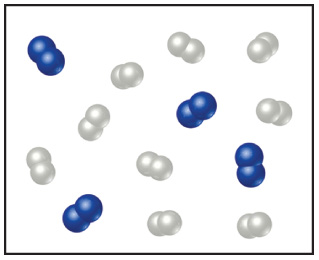# Problem: Nitrogen (N2) and hydrogen (H2) react to form ammonia (NH3). Consider the mixture of N2 and H2 shown in the accompanying diagram. The blue spheres represent N, and the white ones represent H.What is the limiting reactant in this case?

###### FREE Expert Solution

In this problem, we are asked to find which is the limiting reactant in the reaction.

Recall, that the limiting reactant or limiting reagent is the compound that determines the maximum amount of product obtained in a chemical reaction

To solve this problem:

Step 1. Write and balance the chemical reaction
Step 2. Perform a stoichiometric analysis.
Step 3. Identify the limiting reagent.

Step 1. From the problem, the given reaction is:

N2 + H2 → NH3

This equation is not yet balanced. To balance it, we have to make sure that the number of elements on both sides is equal.

91% (371 ratings)###### Problem Details

Nitrogen (N2) and hydrogen (H2) react to form ammonia (NH3). Consider the mixture of N2 and H2 shown in the accompanying diagram. The blue spheres represent N, and the white ones represent H.What is the limiting reactant in this case?